## A bugle can be thought of as an open pipe. If a bugle were straightened out, it would be 2.65 mlong.a.) If the speed of sound is 343m/????,

Question

A bugle can be thought of as an open pipe. If a bugle were straightened out, it would be 2.65 mlong.a.) If the speed of sound is 343m/????, find the lowest frequency that is resonant for a bugle (ignoring end corrections)b.) Find the next two resonant frequencies for the bugle.

in progress 0
2 weeks 2021-09-02T20:07:00+00:00 1 Answers 0 views 0

(a). The lowest frequency is 64.7 Hz.

(b). The next two resonant frequencies for the bugle are 129.4 Hz and 194.2 hz.

Explanation:

Given that,

Length = 2.65 m

Speed of sound = 343 m

We need to calculate the wavelength

Using formula of wavelength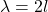Put the value into the formula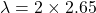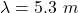(a). We need to calculate the lowest frequency

Using formula of frequency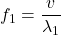Put the value into the formula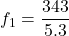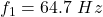(b). We need to calculate the next two resonant frequencies for the bugle

Using formula of resonant frequency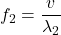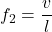Put the value into the formula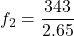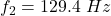For third frequency,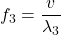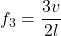Put the value into the formula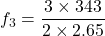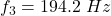Hence, (a). The lowest frequency is 64.7 Hz.

(b). The next two resonant frequencies for the bugle are 129.4 Hz and 194.2 hz.